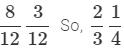Courses

# Test: Fraction - 1

## 10 Questions MCQ Test Mathematics (Maths) for Class 4 | Test: Fraction - 1

Description
This mock test of Test: Fraction - 1 for Class 4 helps you for every Class 4 entrance exam. This contains 10 Multiple Choice Questions for Class 4 Test: Fraction - 1 (mcq) to study with solutions a complete question bank. The solved questions answers in this Test: Fraction - 1 quiz give you a good mix of easy questions and tough questions. Class 4 students definitely take this Test: Fraction - 1 exercise for a better result in the exam. You can find other Test: Fraction - 1 extra questions, long questions & short questions for Class 4 on EduRev as well by searching above.
QUESTION: 1

### What is the fraction used to represent the shaded parts?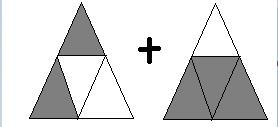Solution:

Number of equal parts = 4

Number of shaded parts = 2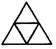Number of equal parts = 4

Number of shaded parts = 3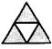So, fraction used to represent the shaded parts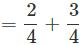QUESTION: 2

### By how much is 19/20 greater than  7/20?

Solution: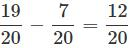QUESTION: 3

###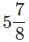is same as ________

Solution:= (5*8 + 7 )/8

=47/8

QUESTION: 4

Which of the following will replace '*'  if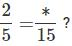Solution: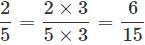QUESTION: 5

How many one-fifths will make one Whole?

Solution:

1/5 * 5 = 1
So, 5 one-fifths will make one whole,

QUESTION: 6

20/15 is same as _______

Solution: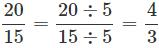QUESTION: 7

If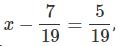then  x = _______

Solution: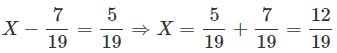QUESTION: 8

The sum of shaded fractions of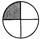and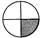is _____.

Solution:

Sum of shaded fractions =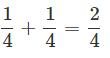QUESTION: 9

Which of the following set of fractions are in descending order?

Solution:
QUESTION: 10

Which of the following options can make the given number sentence true? 2/3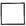1/4

Solution:

Converting the fractions into like fractions. LCM of 3 and 4 = 12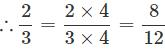and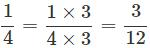Now,